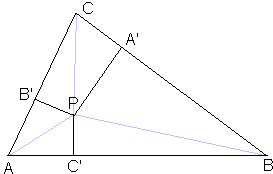# Carnot's Theorem

Carnot's theorem is a direct application of the theorem of Pythagoras. It's a nice little theorem that is unlikely to be rediscovered with dynamic geometry software. A penchant for doodling and imagination this is all that is probably needed to rediscover a theorem like that.Let points $A',$ $B',$ and $C'$ be located on the sides $BC,$ $AC,$ and, respectively, $AB$ of $\Delta ABC.\;$ The perpendiculars to the sides of the triangle at points $A',$ $B',$ and $C'\;$ are concurrent iff

(1)

$AC'^{2} - BC'^{2} + BA'^{2} - CA'^{2} + CB'^{2} - AB'^{2} = 0.$

### Proof

Assume the three perpendiculars meet in point $P.$ The lines $AP,$ $BP,$ $CP,$ $A'P,$ $B'P,$ $C'P$ split the triangle into six right-angled triangles, three pairs of which share a hypotenuse, whilst three other pairs share a leg with a vertex at $P.$ The situation begs for an application of the Pythagorean theorem:

\begin{align} AC'^{2} + C'P^{2} &= AP^{2}\\ BC'^{2} + C'P^{2} &= BP^{2}\\ BA'^{2} + A'P^{2} &= BP^{2}\\ CA'^{2} + A'P^{2} &= CP^{2}\\ CB'^{2} + B'P^{2} &= CP^{2}\\ AB'^{2} + B'P^{2} &= AP^{2}. \end{align}

Taking the first, third, and fifth equations with the sign $"+",$ and the second, fourth, and sixth equations with the sign $"-",$ and adding up all six leads, after obvious simplifications, to (1).

To prove the converse, assume (1) and let $P$ be the point of intersection of, say, $A'P\;$ and $B'P.\;$ Drop a perpendicular $DP\;$ from $P\;$ to $AB.\;$ By the already proven part:

$AD^{2} - BD^{2} + BA'^{2} - CA'^{2} + CB'^{2} - AB^{2} = 0,$

so that

(2)

$AD^{2} - BD^{2} = AC'^{2} - BC'^{2}.$

This is only possible if $D = C'.\;$ Why so? Let $BD = x,$ $AB = c.$ Then $AD = c - x,$ and

$AD^{2} - BD^{2} = (c - x)^{2} - x^{2} = c^{2} - 2cx,$

which is a linear function of x: $f(x) = c^{2} - 2cx.\;$ f therefore is 1-1. In other words, $f(x)$ can't have the same value for two different $x\text{'s}.\;$ This means that (2) does imply $D = C'.$

We are naturally coming to a related problem: Given two points $A\;$ and $B.\;$ What is the locus of points $M\;$ such that $AM^{2} - BM^{2}\;$ is constant?

Let $M$ be a point on the locus and $H\;$ the foot of the perpendicular from $M\;$ to $AB.\;$ By the Pythagorean theorem:

$AM^{2} - AH^{2} = MH^{2} = BM^{2} - BH^{2},$

i.e.,

$AM^{2} - BM^{2} = AH^{2} - BH^{2},$

which means that, if $M$ lies on the locus in question, so does $H.$ And vice versa. Since the same $H$ serves as the foot of the perpendicular $MH$ for all points $M$ of the line perpendicular to $AB$ at $H,$ this line solves the problem.

If we place the origin of a Cartesian coordinates system at $B$ with the $x\text{-axis}\;$ along $AB$ and the $y\text{-axis}\;$ perpendicular to it, we may assign coordinates $(x, y)$ to point $M.\;$ Then

\begin{align} BM^{2} &= x^{2} + y^{2}, \;\text{whereas}\\ AM^{2} &= (c - x)^{2} + y^{2}. \end{align}

Thus, as above,

$AM^{2} - BM^{2} = (c - x)^{2} + y^{2} - x^{2} - y^{2} = f(x),$

where $f(x)$ is the function defined above. If $x$ is fixed, so is $f(x),$ and also $AM^{2} - BM^{2},$ as expected. From this fact, or from the underlying proof, it is clear that, for (1) to hold, the points $A',$ $B',$ $C'$ need not be necessarily located on the sides of $\Delta ABC.$

### Corollary

1. Perpendicular bisectors of the sides of a triangle concur in a point (the circumcenter.)

Indeed, $AC' = BC',$ $BA' = CA',$ and $CB' = AB'$ imply (1).

2. The altitudes of a triangle concur in a point.

Indeed, if $AA',$ $BB',$ and $CC'$ are the altitudes of $\Delta ABC,\;$ then

$AB^{2} - BA'^{2} = AA'^{2} = AC^{2} - CA'^{2},$

so that

$AB^{2} - AC^{2} = BA'^{2} - CA'^{2},$

Similarly,

\begin{align} AC^{2} - BC^{2} &= AC'^{2} - BC'^{2} and\\ BC^{2} - AB^{2} &= CB'^{2} - AB'^{2}, \end{align}

3. The perpendiculars to the sides of $\Delta ABC\;$ at the points where the incircle meets the sides are concurrent (at the incenter.)

Obvious.

4. The perpendiculars to the sides of $\Delta ABC\;$ at the points where the excircles meet the sides are concurrent.

Indeed [F. G.-M., p. 555], in this case we have

$CA' = AC',$ $BA' = AB',$ and $BC' = CB',$

which implies (1).

5. Existence of OrthopoleTo every line m in the plane of \Delta ABC there correspond a point - the orthopole of $M\;$ with respect to $\Delta ABC.\;$ To define the point, first drop perpendiculars $AL,$ $BM,$ and $CN\;$ from the vertices of $\Delta ABC\;$ onto $m.\;$ From the three points thus obtained drop perpendiculars on the "opposite" sides of the triangle: from $L\;$ onto $BC,\;$ from $M\;$ onto $AC,\;$ and from $N\;$ onto $AB.\;$ The latter three lines are concurrent, and the point where they meet is known as the orthopole of $m$ with repsect to $\Delta ABC.$

Darij Grinberg came up with an ingenious proof of this result based on Carnot's theorem (1). As we already know, the points $A',$ $B',$ $C'\;$ need not be located on the sides of $\Delta ABC.\;$ So let's take $A' = L,$ $B' = M,$ and $C' = N.\;$ Applying the Pythagorean theorem several times, we obtain

$AM^{2} - LM^{2} = AL^{2} = AN^{2} - LN^{2},$

so that

$AM^{2} - AN^{2} = LM^{2} - LN^{2}.$

Similarly,

\begin{align} BN^{2} - BL^{2} &= MN^{2} - LM^{2}, \;\text{and}\\ CL^{2} - CM^{2} &= LN^{2} - MN^{2}, \end{align}

$AM^{2} - AN^{2} + BN^{2} - BL^{2} + CL^{2} - CM = 0.$

6. Generalization

Carnot's theorem admits a generalization to an $n\text{-gon},$ with $n\ge 3,$ with the concurrent lines equally slanted to the sides.

### References

1. F. G.-M., Exercices de Géométrie, Éditions Jacques Gabay, sixiéme édition, 1991